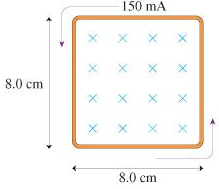# Problem: The resistance of the loop in the figure is 0.30 Ω.a) Is the magnetic field strength increasing or decreasing?b) At what rate (T/s)?

###### FREE Expert Solution
90% (417 ratings)###### Problem Details

The resistance of the loop in the figure is 0.30 Ω.a) Is the magnetic field strength increasing or decreasing?

b) At what rate (T/s)?